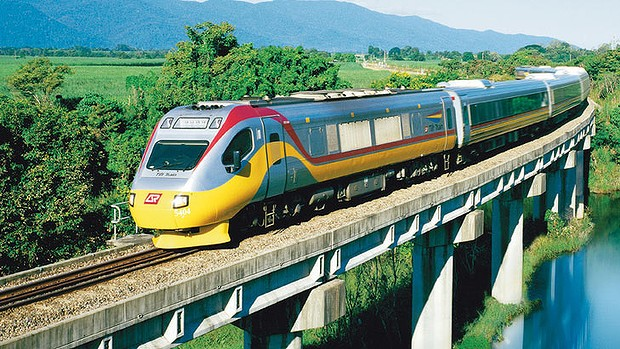# Which side would you want to be in?The two ends of a train moving with uniform acceleration passes a certain point with velocities $12 kmph$ and $15 kmph$ respectively. What is the velocity with which the middle point of the train passes the same point? (Round your answers to nearest hundredth)

×